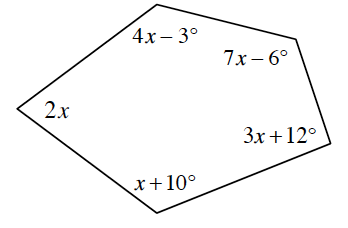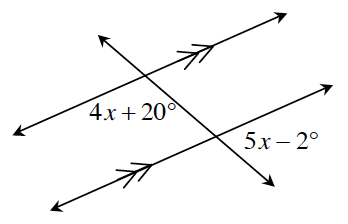### Home > INT2 > Chapter 9 > Lesson 9.3.2 > Problem9-99

9-99.

For each geometric relationship represented below, write and solve an equation for $x$. Show all work.

1.What is the sum of the interior angles of a pentagon?
How can you use this to find $x$?

$x = 31^\circ$, because
$(2x) + (4x − 3) + (7x − 6) + (3x + 12) + (x + 10) = 540^\circ$

1.What is the relationship between these two angles?
Is there an angle that both $4x + 20^\circ\text{ and }5x + 2^\circ$ have a geometric relationship to?

$4x+20^\circ+5x-2^\circ=180^\circ$

$x=18^\circ$